# Math Problem Sets #2 and Solutions To Problem Set One

in STEMGeeks2 years ago

Hello there. Here is the second set of math problems. Solutions to the first one can be found below after the second set of problems.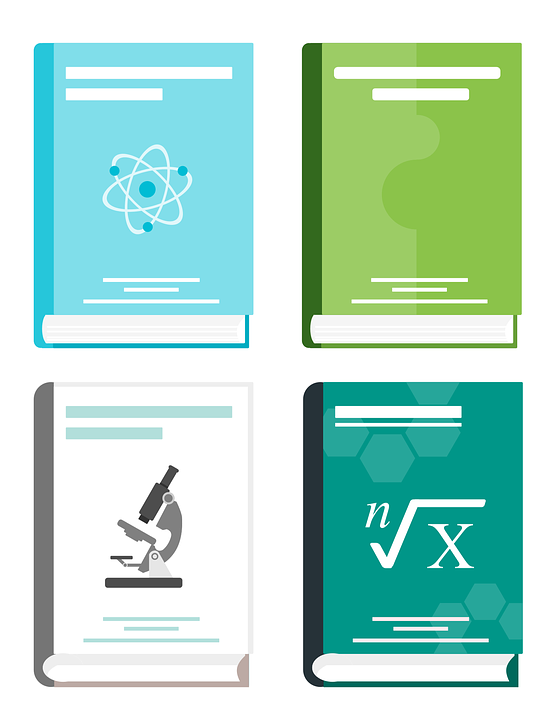Pixabay Image Source

# Math Problem Set Two

Question One

Determine the angle measures of `w` and `v` if `w` is supplementary to angle `v`. In addition, `w` is 18 degrees more than angle `v`.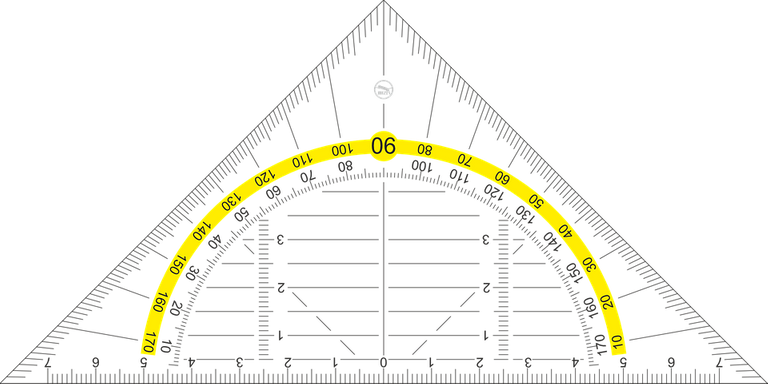Pixabay Image Source

Question Two

There are 9 gold coins. Eight of the nine are real and one is fake. The fake coin is heavier than the real gold coins. Given a balance scale, how can you determine which coin is the fake one?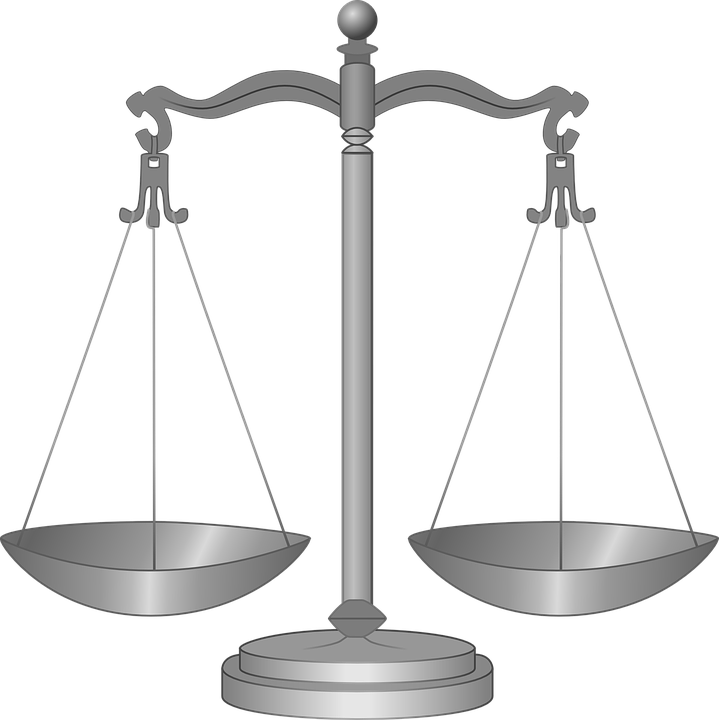Pixabay Image Source

Question Three

There are five strangers who are meeting each other for the first time. Every person shakes each other hand exactly once . How many handshakes occur?

You may use a diagram or a table as assistance.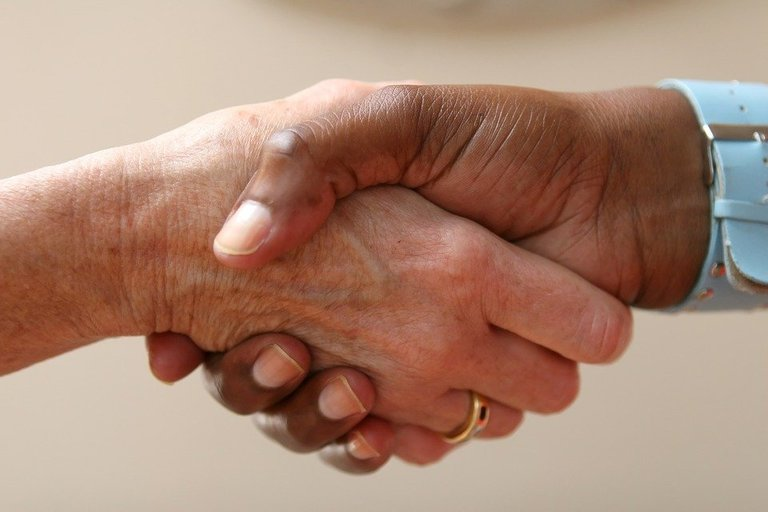Pixabay Image Source

# Solutions To Math Problem Set One

Question One

A square is a rectangle. Explain why a rectangle is not a square.

Solution

A square is a four sided shape where all the side lengths are the same, opposite sides are parallel and equal and the corners/vertices are at 90 degree angles.

A rectangle is a four sided shape where opposite side lengths are the same and are parallel. The corners of a rectangle contain 90 degree angles.

Squares contains everything from rectangles. A rectangle is not a square as all the sides are not equal.

Question Two

What is the perimeter of a square with an area of 100 square centimetres?

Solution

The area of a square is the side length multiplied by itself. With an area of 100 square centimetres for a square, the side length is 10 centimetres.

A square has all four sides being the same length. The perimeter for this square is 10cm + 10cm + 10cm + 10cm or 4 x 10 cm = 40cm.

Question Three

What is the volume of a cylinder with a diameter of 2Π cm and a height of 10 cm?

Solution

Diameter of a circle is twice the radius. The radius here is Π (pi) centimetres.

The volume of the cylinder is the area of the circle multiplied by the height.

The area of the circle is `Π * r * r` which is `Π * Π * Π` or Π cubed.

Combining Π cubed multiplied by the height of 10 centimetres gives the volume (product) of 10 Π cubed cubic centimetres.

Question Four

Three consecutive odd numbers add up to 51. What are these odd numbers?

Solution

Denote `y` as the first odd number in the three number sequence.

These three consecutive odd numbers show up as `y, y +2, y + 4`. These three odd numbers add up to 51. This can be expressed as this equation below.

`y + (y + 2) + (y +4) = 51`

`3y + 6 = 51`

`3y = 45`

`y = 15`

Fifteen is the first odd number. It follows that the next two odd numbers are 17 and 19. You can check that 15, 17 and 19 add up to 51.

Question Five

What is the probability of a fair coin landing heads and rolling a fair six sided die that is a factor of 6?

Solution

Landing heads with a fair coin has a probability of a half or 0.5.

Factors of 6 are 6, 2, 3 and 1. These are four numbers from the six sided die. Rolling a fair six sided die that is a factor of 6 is 4 out of 6 or 2 out of 3.

The fractions 1/2 and 2/3 can be multiplied together (independent events that have no effect on each other on number of outcomes) to obtain 1/3.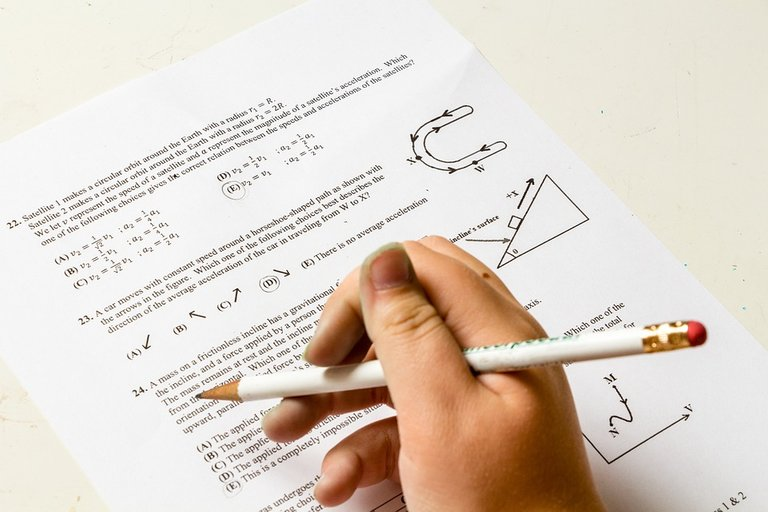Pixabay Image Source